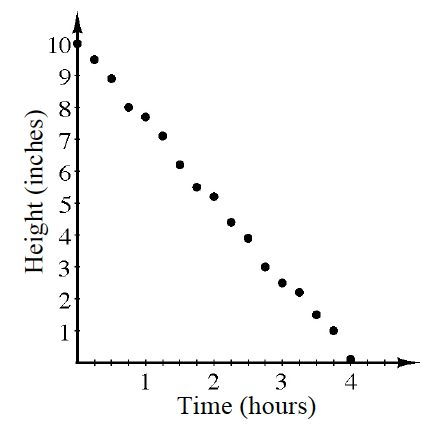### Home > INT1 > Chapter 3 > Lesson 3.3.1 > Problem3-119

3-119.At an aunt’s wedding, Nicolas collected data about an ice sculpture that was about to completely melt. A graph of his data is shown at right.

1. Write an equation for a line of best fit. What are the units for the slope and $y$-intercept in the equation?

• Use $y = mx + b$.

1. Based on your equation, how tall was the ice sculpture one hour before Nicolas started measuring?

$12.5$ inches